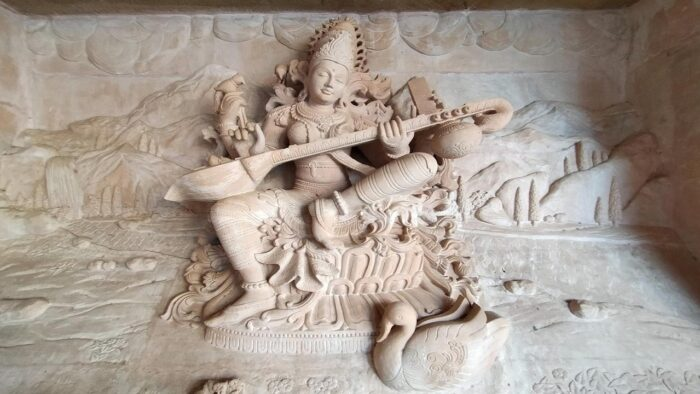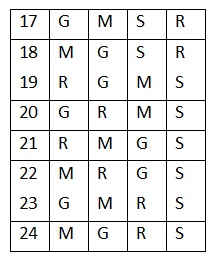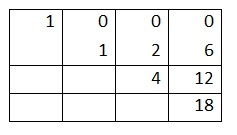# Sarngadeva’s Taana Prastaar – Part IIIThis is in continuation of my earlier articles – Sarngadeva’s Taana Prastaar, Part 1 and  Part 2.

Given a row number of a taana prastaar can we generate the arrangement of the svaras in the taana?

Let us find the arrangement of the svaras in the 21st row of a 4-svara taana prastaar containing the svaras S R G M.

A 4-svara taana prastaar will contain 4! = 24 rows / taanas.

A 4-svara taana prastaar will contain four 3-svara prastaars. These four 3-svara taana prastaars will be appended by the svaras in the following order – M G R S. A 3-svara taana prastaar will have 3! = 6 rows / taanas. The multiples of 6 are 0, 6, 12 and 18. The highest multiple which is less than n = 21 (the given row number) is 18. Hence, the rank is 4. Thus the last svara of 21st row will be S. We now have, n = 21 – 18 = 3.

A 3-svara taana prastaar will contain three 2-svara prastaars. These three 2-svara taana prastaars will be appended by the svaras in the following order – M G R. A 2-svara taana prastaar will have 2! = 2 rows / taanas. The multiples of 2 are 0, 2 and 4. The highest multiple which is less than n = 3 is 2. Hence, the rank is 2. Thus the 3rd svara of 21st row will be G. We now have, n = 3 – 2 = 1.

A 2-svara taana prastaar will contain two 1-svara prastaars. These two 1-svara taana prastaars will be appended by the svaras in the following order – M R. A 1-svara taana prastaar will have only 1 row / taana. The multiples of 1 are 0 and 1. The highest multiple which is less than n = 1 is 0. Hence, the rank is 1. Thus the 2rd svara of 21st row will be M. We now have, n = 1 – 0 = 1.

Now, R is only svara that is remaining. Hence, the first svara of 21st row will be R.

Hence the 21st taana in a 4-svara taana prastaar will be R M G S.

Verify the answer by looking it up in the 4-svara taana prastaar given in Table 1.Table 1

यैरङ्कैर्नष्टसङ्ख्या स्यान्मौलैकाङ्कसमन्वितैः॥

तेषु लोष्टं क्षिपेन्मूले लोष्टस्थानमितं भवेत्।

नष्टतानस्वरस्थानं ततो यावतिथे पदे॥

अधःक्रमादस्तिलोष्टः स्वरस्तावतिथो भवेत्।

क्रमान्तिमस्वरात्पूर्वो लब्धत्यागादि पूर्ववत्॥   (सङ्गीतरत्नाकरः १.४.६८-७०)

• To find the taana (of n svaras) corresponding to a given row-number (nashta-sankhya), mark the entry just below the row number in the n-th column of the Khandmeru.
• Subtract that entry from the row number and mark the entry, which is just below the resulting number, in the next or the (n – 1)-th column. And so on.
• The position of the marked entry in the last column gives the rank of the last svara of the taana in the reverse of the natural order.
• The position of the marked entry in the next column gives the rank of the second last svara, amongst the remaining svaras, in the reverse of the natural order. And so on.

Find the 19th taana in the prastaar of S R G M.

In the fourth column of the Khandmeru, the number just below 19 is 18, which is in the 4th row. Refer Table 2. Hence, the fourth svara is the last among SRGM in the reverse order: STable 2

19 – 18 = 1. In the third column, the number just below 1 is 0, which is in the first row. Hence, the next svara is the first among RGM in reverse order: M

1 – 0 = 1. In the second column, the number just below 1 is 0, which is in the first row. Hence the next svara is the first among RG in reverse order: G

1 – 0 = 1. The only svara left is R

The 19th taana is R G M S

Verify the answer by looking it up in Table 1.

Sarngadeva was amongst the earliest to develop sophisticated algorithms for combinatorial sequence generation.

This concludes the series of articles on Sarngadeva’s Taana prastaar.

Feature Image Credit:picxy.com

Disclaimer: The opinions expressed in this article belong to the author. Indic Today is neither responsible nor liable for the accuracy, completeness, suitability, or validity of any information in the article.